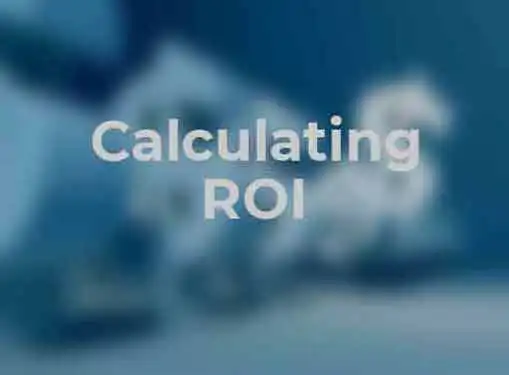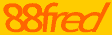# Calculating ROI

The principle behind return on investment (ROI) analysis is straightforward. But calculating ROI can be trickier than it sounds, especially if you aren't used to incorporating quantified metrics into your decision-making processes.

ROI analysis is a mainstay of business decision-making.Businesses that need to make every dollar count (and what business doesn't?) use ROI to quantify the potential outcomes of their investment decisions and to maximize the profits they receive from the choices they make. When applied consistently, ROI methodology can help you develop a more confident, more decisive style of leadership.

At a glance, calculating ROI looks like a simple and straightforward process. While it's true that you don't need a Ph.D. in math to perform accurate ROI analysis, the process can actually become much more sophisticated than it seems.

The application of a standard formula is only one part of ROI analysis. Of equal importance are the accuracy of the inputs and the manner in which you apply ROI to decision-making processes. Sometimes you will discover that the ROI calculation for a decision that seemed to be a no-brainer loses its luster when compared to other alternatives.

If the consistent application of ROI analysis is new to you, here are the basic steps involved with calculating ROI.

• Quantify Benefits. The first step in calculating ROI is to quantify the potential benefits of your decision. If the benefit is a dollar value, Step 1 is a piece of cake. But what if the benefit is based on a different unit of measurement? For example, what if the benefit you hope to achieve is a 10% increase in productivity? For the purpose of ROI analysis, you will need to convert "productivity" into a dollar value or other unit of measurement.
• Identify Costs. The next step is to identify the cost needed to achieve the desired benefit, expressed in the same unit of measurement as the benefit. If a 10% increase in productivity can be translated into an annual benefit of \$50,000, then the cost will be expressed in a dollar value. But if for some reason it's more useful to express the benefit as 50,000 additional widgets, the cost should be translated into widgets, as well.
• Apply ROI Formula. After you have determined benefit and cost values, you can apply the basic ROI formula: (Benefit-Cost)/Cost. So if the benefit value is \$50,000 and the cost value is \$40,000, ROI would be (\$50,000-\$40,000)/\$40,000 or .25.
• Determine ROI Percentage. The final step in calculating ROI is to multiply this figure by 100 to convert it to a percentage. When applied to the previous example, ROI would be 25%. Not astronomical, but not bad compared to letting your money lie dormant in a savings account or CD.

## Conversation Board

We greatly appreciate any advice you can provide on this topic. Please contribute your insights on this topic so others can benefit.